# Objective

The objectives for this week are:

• Understand the the process of boosting to improve model accuracy
• Learn about fitting a support vector machine model
• Examine the differences between results from different models

# Class discussion

This is a diagram explaining boosting. The three tree models in the top row are combined to give the boosted model in box 4. Come up with a some words and sentences, together, to explain the process.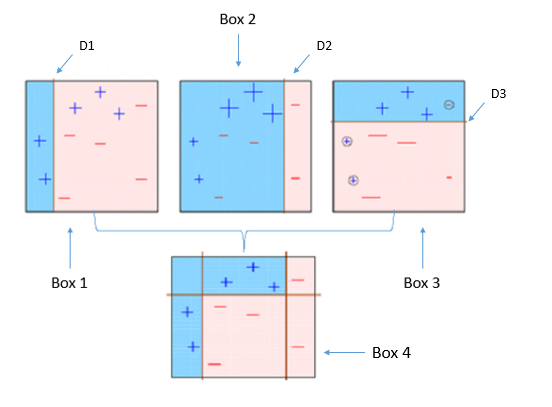How would a single tree with multiple splits fit this data? What is different about the two approaches?

# Theory

Fill in the steps to go from the first line to the last

\begin{align*} \mathcal{K}(\mathbf{x}, \mathbf{y}) & = (1 + \langle \mathbf{x}, \mathbf{y}\rangle) ^2 \\ & = ??? \\ & = ???\\ & = ???\\ & = (1, x_1^2, x_2^2, \sqrt2x_1, \sqrt2x_2, \sqrt2x_1x_2)^T(???) \\ & = \langle \psi(\mathbf{x}), \psi(\mathbf{y}) \rangle \end{align*}

# Practice

## 1.

Fit the linear SVM to olive oils, using a training split of 2/3, using only regions 2, 3, and the predictors linoleic and arachidic.

1. Report the training and test error,
2. list the support vectors,
3. the coefficients for the support vectors and
4. the equation for the separating hyperplane, and $???\times\text{linoleic}+???\times\text{arachidic}+??? > 0$
5. make a plot of the boundary.
6. Write a paragraph explaining how this model fit differs from the tree, and random forests fit the data in the last lab.

## 2.

Fit the SVM again to the full set of variables. Generate the predictions from this model for your gridded data, and plot them for linoleic and arachidic acid. You will need to set some fixed value for the other variables, say the mean, so that the gridded data has all variables. Explain how the boundary changes, if is does.

## 3.

This last question revisits the paintings problem, to see how random forests compares with a boosted tree model, on really tough problem.

The purpose is to automatically analyse the happy paintings by Bob Ross. This was the subject of the 538 post, “A Statistical Analysis of the Work of Bob Ross”.

We have taken the painting images from the sales site, read the images into R, and resized them all to be 20 by 20 pixels. Each painting has been classified into one of 8 classes based on the title of the painting. This is the data that you will work with.

It is provided in wide and long form. Long form is good for making pictures of the original painting, and the wide form is what you will need to use for fitting the classification models. In wide form, each row corresponds to one painting, and the rgb color values at each pixel are in each column. With a $$20\times20$$ image, this leads to $$400\times3=1200$$ columns.

Here are three of the original paintings in the collection, labelled as “scene”, “water”, “flowers”: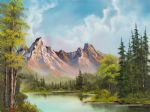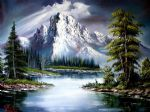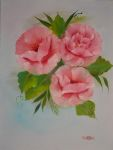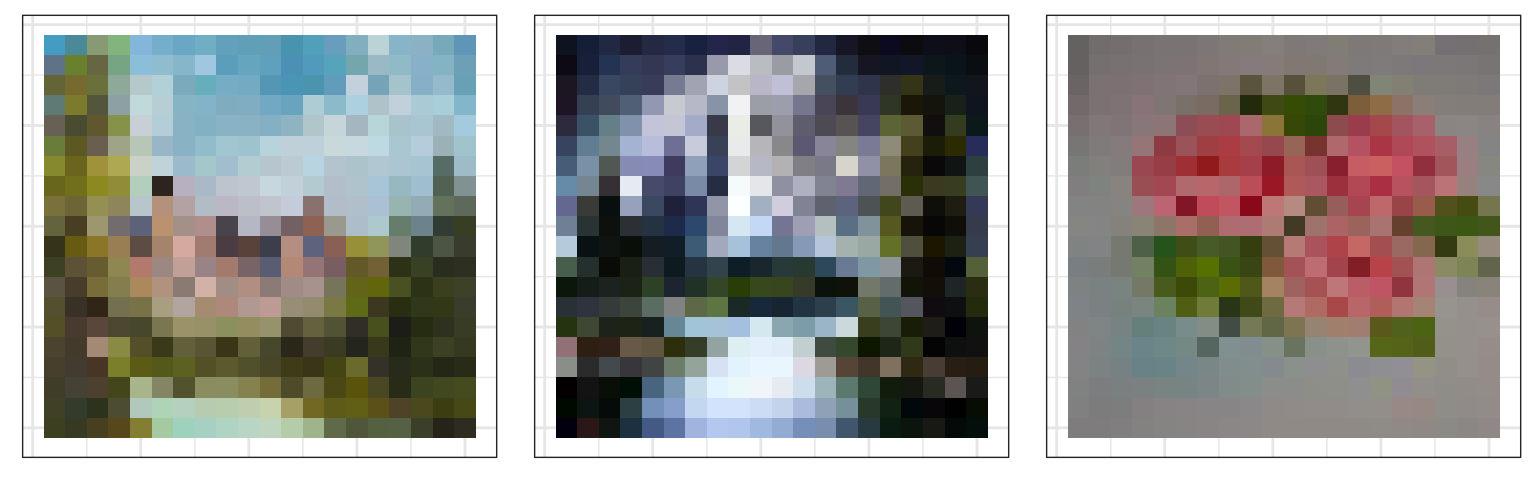1. Build a random forest for the training data, for two classes, flowers and water. Predict the class of test set, report the error.

2. Read the description of the XGBoost technique at https://www.hackerearth.com/practice/machine-learning/machine-learning-algorithms/beginners-tutorial-on-xgboost-parameter-tuning-r/tutorial/, or other sources. Tune the model fit to determine how many iterations to make. Then fit the model, using the parameter set provided. Fit the xgboost model to the paintings data. Compute the error for the test set, and describe the difference with the results from the random forest.